This case study uses Stan to fit the Rating Scale Model (RSM) and Generalized Rating Scale Model (GRSM), including a latent regression for person ability for both. Analysis is performed with R, making use of the rstan and edstan packages. rstan is the implementation of Stan for R, and edstan provides Stan models for item response theory and several convenience functions.

The edstan package is available on CRAN, but a more up to date version may often be found on Github. The following R code may be used to install the package from Github.

# Install edstan from Github rather than CRAN
install.packages("devtools")
devtools::install_github("danielcfurr/edstan")

The following R code loads the necessary packages and then sets some rstan options, which causes the compiled Stan model to be saved for future use and the MCMC chains to be executed in parallel.

# Load R packages
library(rstan)
library(ggplot2)
library(edstan)
library(ltm)
rstan_options(auto_write = TRUE)
options(mc.cores = parallel::detectCores())

The case study uses R version 3.3.2, rstan version 2.14.1, ggplot2 version 2.2.1, and edstan version 1.0.7. Also, the example data are from TAM version 1.0.0. Readers may wish to check the versions for their installed packages using the packageVersion() function.

# 1 Rating scale model with latent regression

## 1.1 Overview of the model

The rating scale model (Andrich 1978) is appropriate for item response data that involves Likert scale responses. The version presented includes a latent regression. However, the latent regression part of the model may be restricted to an intercept only, resulting in the standard rating scale model.

$\Pr(Y_{ij} = y,~y > 0 | \theta_j, \beta_i, \kappa_s) = \frac{\exp \sum_{s=1}^y (\theta_j - \beta_i - \kappa_s)} {1 + \sum_{k=1}^{m} \exp \sum_{s=1}^k (\theta_j - \beta_i - \kappa_s)}$ $\Pr(Y_{ij} = y,~y = 0 | \theta_j, \beta_i, \kappa_s) = \frac{1} {1 + \sum_{k=1}^{m} \exp \sum_{s=1}^k (\theta_j - \beta_i - \kappa_s)}$ $\theta_j \sim \mathrm{N}(w_{j}' \lambda, \sigma^2)$

Variables:

• $$i = 1 \ldots I$$ indexes items.
• $$j = 1 \ldots J$$ indexes persons.
• $$Y_{ij} \in \{ 0 \ldots m \}$$ is the response of person $$j$$ to item $$i$$
• $$m$$ is simultaneously the maximum score and number of step difficulty parameters per item.
• $$w_{j}$$ is the vector of covariates for person $$j$$, the first element of which must equal one for a model intercept. $$w_{j}$$ may be assembled into a $$J$$-by-$$K$$ covariate matrix $$W$$, where $$K$$ is number of elements in $$w_j$$.

Parameters:

• $$\beta_i$$ is the item-specific difficulty for item $$i$$.
• $$\kappa_s$$ is the $$s$$-th step difficulty, constant across items.
• $$\theta_j$$ is the ability for person $$j$$.
• $$\lambda$$ is a vector of latent regression parameters of length $$K$$.
• $$\sigma^2$$ is the variance for the ability distribution.

Constraints:

• The last item difficulty parameter, $$\beta_I$$, is constrained to be the negative sum of the other difficulties, resulting in the average item difficulty parameter being zero.
• The last step difficulty parameter, $$\kappa_m$$, is likewise constrained to be the negative sum of the other step difficulties, resulting in the average step difficulty being zero.

Priors:

• $$\sigma \sim \mathrm{Exp}(.1)$$ is weakly informative for the person standard deviation.
• $$\beta_i \sim \mathrm{N}(0, 9)$$ is also weakly informative.
• $$\kappa_s \sim \mathrm{N}(0, 9)$$ is weakly informative.
• $$\lambda \sim t_3(0, 1)$$, where $$t_3$$ is the Student’s $$t$$ distribution with three degrees of freedom, and the covariates have been transformed as follows: (1) continuous covariates are mean-centered and then divided by two times their standard deviations, (2) binary covariates are mean-centered and divided their maximum minus minimum values, and (3) no change is made to the constant, set to one, for the model intercept. This approach to setting priors is similar to one that has been suggested for logistic regression (Gelman et al. 2008). It is possible to adjust the coefficients back to the scales of the original covariates.

## 1.2Stan code for a simple rating scale model

A simple Stan model is described before discussing the complete model, as the code for the complete model is somewhat cumbersome. The simpler model, printed below, omits the latent regression and so does not require rescaling of the person covariates or lambda. The mean of the person distribution is set to zero and the constraint is removed from the item difficulties, which also differs from the complete model.

# Print the simple RSM from the edstan package
simple_rsm_file <- system.file("extdata/rsm_simple.stan",
package = "edstan")
cat(readLines(simple_rsm_file), sep = "\n")
functions {
real rsm(int y, real theta, real beta, vector kappa) {
vector[rows(kappa) + 1] unsummed;
vector[rows(kappa) + 1] probs;
unsummed = append_row(rep_vector(0, 1), theta - beta - kappa);
probs = softmax(cumulative_sum(unsummed));
return categorical_lpmf(y + 1 | probs);
}
}
data {
int<lower=1> I;                // # items
int<lower=1> J;                // # persons
int<lower=1> N;                // # responses
int<lower=1,upper=I> ii[N];    // i for n
int<lower=1,upper=J> jj[N];    // j for n
int<lower=0> y[N];             // response for n; y in {0 ... m_i}
}
transformed data {
int m;                         // # steps
m = max(y);
}
parameters {
vector[I] beta;
vector[m-1] kappa_free;
vector[J] theta;
real<lower=0> sigma;
}
transformed parameters {
vector[m] kappa;
kappa[1:(m-1)] = kappa_free;
kappa[m] = -1*sum(kappa_free);
}
model {
beta ~ normal(0, 3);
target += normal_lpdf(kappa | 0, 3);
theta ~ normal(0, sigma);
sigma ~ exponential(.1);
for (n in 1:N)
target += rsm(y[n], theta[jj[n]], beta[ii[n]], kappa);
}

The functions block includes a user-specified function rsm(), which accepts a response y, a value for theta, a scalar beta for one item, and the vector kappa. With these inputs, it returns the model-predicted log probability for the response. Later, in the model block, rsm() is used to get the likelihood of the observed item responses.

Looking to the data block, data are fed into the model in vector form. That is, y is a long vector of scored item responses, and ii and jj indicate with which item and person each element in y is associated. These three vectors are of length N, which is either equal to I times J or less if there are missing responses. Then in the transformed data block, the variable m is created, which represents the number of steps per item.

In the parameters block, kappa_free is declared as a vector of length m - 1 and represents the unconstrained step parameters. In the transformed parameters block, the constrained step parameter is appened to kappa_free to make kappa, which is the complete vector of step difficulties. The other parameters are handled in conventional ways, with sigma being assigned a lower bound of zero because it is a standard deviation.

The model block indicates the priors and the likelihood. The target += ... syntax for the prior on kappa is a manual way of incrementing the log posterior used when the prior is placed on a transformed parameter. The likelihood uses similar syntax along with the rsm() function.

## 1.3Stan code for the rating scale model with latent regression

The RSM with latent regression will be discussed in relation to the simpler model, and both models are equivalent when the latent regression is restricted to an intercept only. The model with latent regression, which is featured in edstan, is printed below. It is more complicated than is typically necessary for a Stan model because it is written to apply sensible priors automatically for parameters associated with arbitrarily scaled covariates.

functions {
real rsm(int y, real theta, real beta, vector kappa) {
vector[rows(kappa) + 1] unsummed;
vector[rows(kappa) + 1] probs;
unsummed = append_row(rep_vector(0, 1), theta - beta - kappa);
probs = softmax(cumulative_sum(unsummed));
return categorical_lpmf(y + 1 | probs);
}
matrix obtain_adjustments(matrix W) {
real min_w;
real max_w;
int minmax_count;
matrix[2, cols(W)] adj;
adj[1, 1] = 0;
adj[2, 1] = 1;
if(cols(W) > 1) {
for(k in 2:cols(W)) {                       // remaining columns
min_w = min(W[1:rows(W), k]);
max_w = max(W[1:rows(W), k]);
minmax_count = 0;
for(j in 1:rows(W))
minmax_count = minmax_count + W[j,k] == min_w || W[j,k] == max_w;
if(minmax_count == rows(W)) {       // if column takes only 2 values
adj[1, k] = mean(W[1:rows(W), k]);
adj[2, k] = (max_w - min_w);
} else {                            // if column takes > 2 values
adj[1, k] = mean(W[1:rows(W), k]);
adj[2, k] = sd(W[1:rows(W), k]) * 2;
}
}
}
return adj;
}
}
data {
int<lower=1> I;                // # items
int<lower=1> J;                // # persons
int<lower=1> N;                // # responses
int<lower=1,upper=I> ii[N];    // i for n
int<lower=1,upper=J> jj[N];    // j for n
int<lower=0> y[N];             // response for n; y in {0 ... m_i}
int<lower=1> K;                // # person covariates
matrix[J,K] W;                 // person covariate matrix
}
transformed data {
int m;                         // # steps
matrix[2,K] adj;               // values for centering and scaling covariates
matrix[J,K] W_adj;             // centered and scaled covariates
m = max(y);
adj = obtain_adjustments(W);
for(k in 1:K) for(j in 1:J)
W_adj[j,k] = (W[j,k] - adj[1,k]) / adj[2,k];
}
parameters {
vector[I-1] beta_free;
vector[m-1] kappa_free;
vector[J] theta;
real<lower=0> sigma;
vector[K] lambda_adj;
}
transformed parameters {
vector[I] beta;
vector[m] kappa;
beta[1:(I-1)] = beta_free;
beta[I] = -1*sum(beta_free);
kappa[1:(m-1)] = kappa_free;
kappa[m] = -1*sum(kappa_free);
}
model {
target += normal_lpdf(beta | 0, 3);
target += normal_lpdf(kappa | 0, 3);
theta ~ normal(W_adj*lambda_adj, sigma);
lambda_adj ~ student_t(3, 0, 1);
sigma ~ exponential(.1);
for (n in 1:N)
target += rsm(y[n], theta[jj[n]], beta[ii[n]], kappa);
}
generated quantities {
vector[K] lambda;
lambda[2:K] = lambda_adj[2:K] ./ to_vector(adj[2,2:K]);
lambda = W_adj[1, 1:K]*lambda_adj[1:K] - W[1, 2:K]*lambda[2:K];
}

The complete model adds obtain_adjustments() to the functions block, which is used to adjust the covariate matrix. In brief, the model operates on the adjusted covariate matrix, W_adj, and then in the generated quantities block determines what the latent regression coefficients would be on the original scale of the covariates. For a more in depth discussion of obtain_adjustments() and the transformations related to the latent regression, see the Rasch and 2PL case study.

In the data block, the number of covariates (plus the intercept) K is now required, as is the matrix of covariates W. The parameters beta_free, kappa_free, theta, sigma, and lambda are declared in the parameters block. The unconstrained item parameters are contained in beta_free. In the transformed parameters block, beta is created by appending the constrained item difficulty to beta_free. The model block contains the priors and the likelihood.

## 1.4 Simulation for parameter recovery

The Stan model is fit to a simulated dataset to evaluate it’s ability to recover the generating parameter values. The R code that follows simulates a dataset conforming to the model.

# Set parameters for the simulated data
J <- 500
sigma <- 1.2
lambda <- c(-10*.05, .05, .5, -.025)
w_2 <- rnorm(J, 10, 5)
w_3 <- rbinom(J, 1, .5)
W <- cbind(1, w_2, w_3, w_2*w_3)

# Set item parameters
I <- 20  # Number of items
S <- 5   # Number of response catetories
beta <- seq(from = -1, to = 1, length.out = I)
kappa <- seq(from = -1, to = 1, length.out = S - 1)

# A function to simulate responses from the model
simulate_response <- function(theta, beta, kappa) {
unsummed <- c(0, theta - beta - kappa)
numerators <- exp(cumsum(unsummed))
denominator <- sum(numerators)
response_probs <- numerators/denominator
simulated_y <- sample(1:length(response_probs) - 1, size = 1,
prob = response_probs)
return(simulated_y)
}

# Calculate or sample remaining variables and parameters
N <- I*J
ii <- rep(1:I, times = J)
jj <- rep(1:J, each = I)
rsm_theta <-  rnorm(J, W %*% matrix(lambda), sigma)
rsm_y <- numeric(N)
for(n in 1:N) {
rsm_y[n] <- simulate_response(rsm_theta[jj[n]], beta[ii[n]], kappa)
}

# Assemble the data list using an edstan function
sim_rsm_list <- irt_data(y = rsm_y, ii = ii, jj = jj,
covariates = as.data.frame(W),
formula = NULL)

The simulated data consists of 20 items having 3 response categories and 500 persons. The person covariate vectors $$w_j$$ include (1) a value of one for the model intercept, (2) a random draw from a normal distribution with mean of 10 and standard deviation of 5, (3) an indicator variable taking values of zero and one, and (4) an interaction between the two. These are chosen to represent a difficult case for assigning automatic priors for the latent regression coefficients. The generating coefficients $$\lambda$$ for the latent regression are -0.5, 0.05, 0.5, and -0.025. The abilities $$\theta$$ are random draws from a normal distribution with a mean generated from the latent regression and a standard deviation $$\sigma = 1.2$$.

# Plot mean ability conditional on the covariates
f1 <- function(x) lambda + x*lambda
f2 <- function(x) lambda + lambda + x*(lambda + lambda)
ggplot(data.frame(w2 = c(0, 20))) +
aes(x = w2) +
stat_function(fun = f1, color = "red") +
stat_function(fun = f2, color = "blue") +
ylab("Mean generated ability") +
xlab("Value for continous covariate")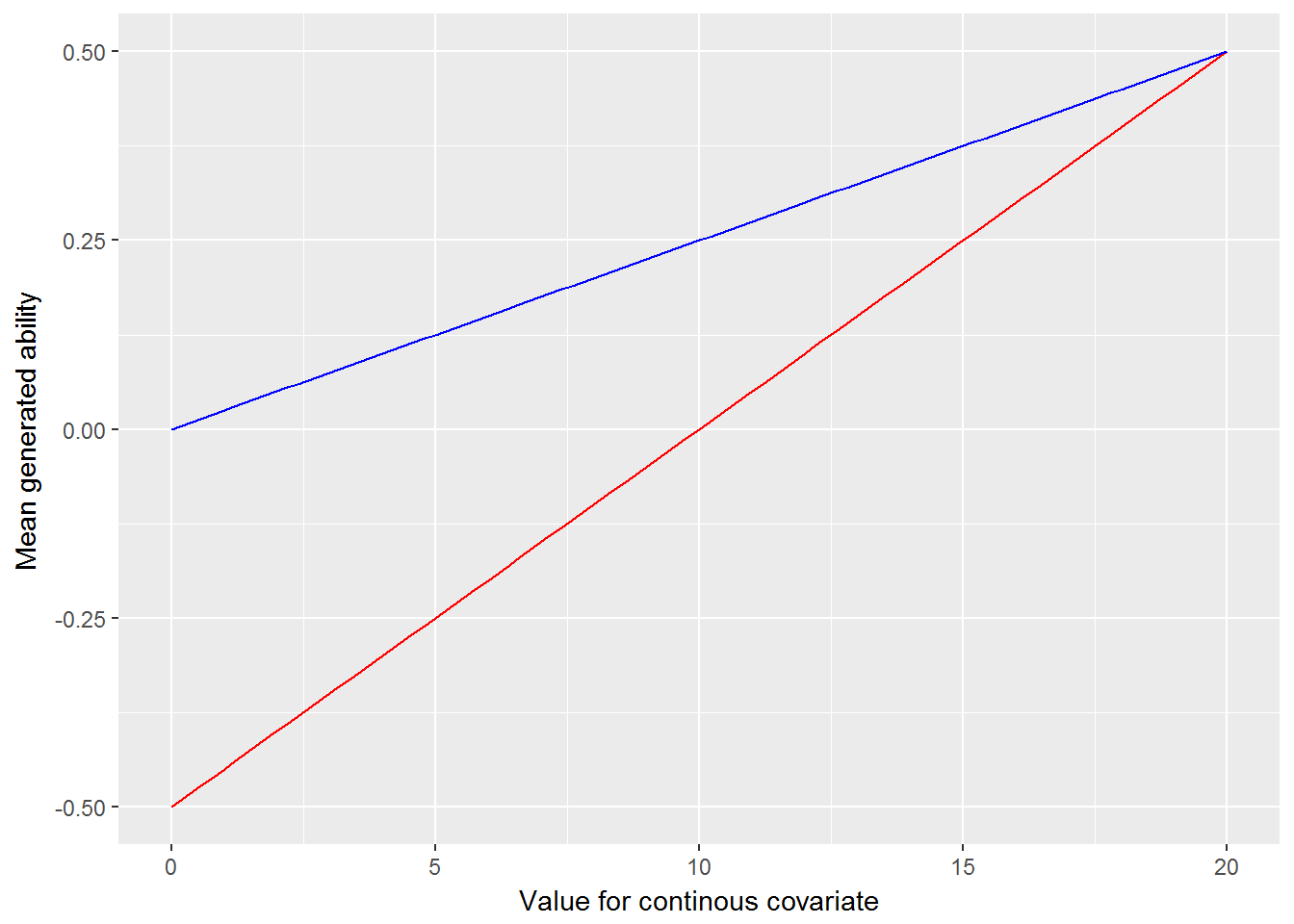Mean of generated abilities as a function of the continuous covariate. A line is shown separately for the two groups identified by the binary variable.

The simulated dataset is next fit with Stan using irt_stan() from the edstan package. irt_stan() is merely a wrapper for stan() in rstan. Using 1,000 posterior draws per chain may be somewhat excessive as we are mainly interested in the posterior means of the parameters. However, as parameter recovery will be evaluated using the 2.5th and 97.5th percentiles of the posterior, the large number of posterior samples is warranted.

#Fit model to simulated data
sim_rsm_fit <- irt_stan(sim_rsm_list, model = "rsm_latent_reg.stan",
chains = 4, iter = 1000)

The highest value for $$\hat R$$ was 1.004 for all parameters and the log posterior, suggesting that the chains have converged. The Stan model is evaluated in terms of its ability to recover the generating values of the parameters. The R code below prepares a plot in which the points indicate the difference between the posterior means and generating values for the parameters of main interest. This difference is referred to as discrepancy. The lines indicate the 95% poster intervals for the difference, defined as the 2.5th and 97.5th percentiles of the posterior draws. Ideally, (nearly) all the 95% intervals would include zero.

# Get estimated and generating values for wanted parameters
rsm_generating_values <- c(beta, kappa, lambda, sigma)
rsm_estimated_values <- summary(sim_rsm_fit,
pars = c("beta", "kappa", "lambda", "sigma"),
probs = c(.025, .975))
rsm_estimated_values <- rsm_estimated_values[["summary"]]

# Make a data frame of the discrepancies
rsm_discrep <- data.frame(par = rownames(rsm_estimated_values),
mean = rsm_estimated_values[, "mean"],
p025 = rsm_estimated_values[, "2.5%"],
p975 = rsm_estimated_values[, "97.5%"],
gen = rsm_generating_values)
rsm_discrep$par <- with(rsm_discrep, factor(par, rev(par))) rsm_discrep$lower <- with(rsm_discrep, p025 - gen)
rsm_discrep$middle <- with(rsm_discrep, mean - gen) rsm_discrep$upper <- with(rsm_discrep, p975 - gen)

# Plot the discrepancies
ggplot(rsm_discrep) +
aes(x = par, y = middle, ymin = lower, ymax = upper) +
scale_x_discrete() +
labs(y = "Discrepancy", x = NULL) +
geom_abline(intercept = 0, slope = 0, color = "white") +
geom_linerange() +
geom_point(size = 2) +
theme(panel.grid = element_blank()) +
coord_flip()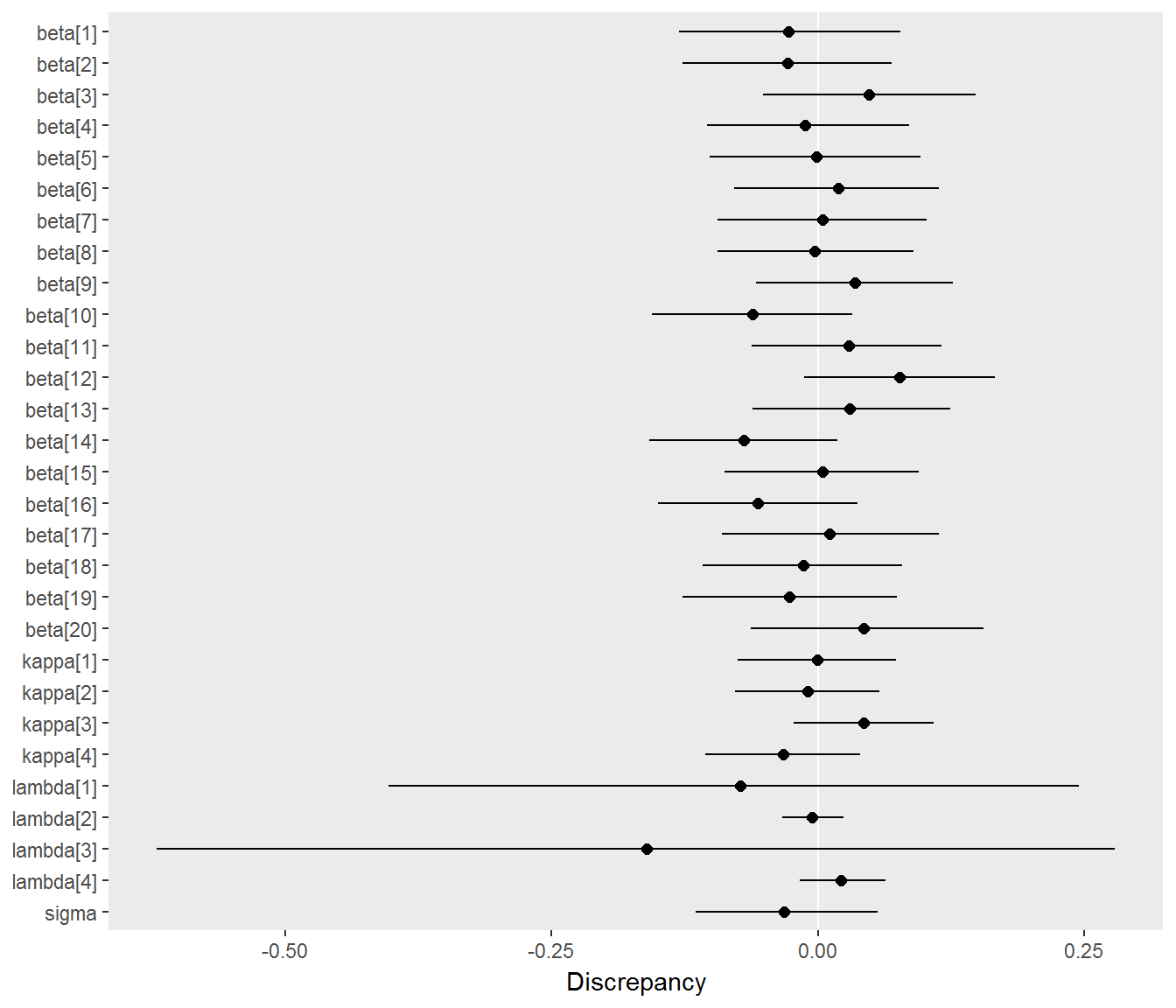Discrepancies between estimated and generating parameters. Points indicate the difference between the posterior means and generating values for a parameter, and horizontal lines indicate 95% posterior intervals for the difference. Most of the discrepancies are about zero, indicating that Stan successfully recovers the true parameters.

# 2 Generalized rating scale model with latent regression

## 2.1 Overview of the model

The GRSM extends the RSM by including a discrimination term. The version presented includes a latent regression. However, the latent regression may be restricted to a model intercept, resulting in the standard generalized rating scale model.

$\Pr(Y_{ij} = y,~y > 0 | \theta_j, \alpha_i, \beta_i, \kappa_s) = \frac{\exp \sum_{s=1}^y (\alpha_i \theta_j - \beta_i - \kappa_s)} {1 + \sum_{k=1}^{m} \exp \sum_{s=1}^k (\alpha_i \theta_j - \beta_i - \kappa_s)}$ $\Pr(Y_{ij} = y,~y = 0 | \theta_j, \alpha_i, \alpha_i, \beta_i) = \frac{1} {1 + \sum_{k=1}^{m} \exp \sum_{s=1}^k (\alpha_i \theta_j - \beta_i - \kappa_s)}$ $\theta_j \sim \mathrm{N}(w_{j}' \lambda, 1)$

Many aspects of the GRSM are similar to the RSM described earlier. Parameters $$\beta_i$$, $$\kappa_s$$, $$\theta_j$$, and $$\lambda$$ have the same interpretation, but the GRSM adds a discrimination parameter $$\alpha_i$$ and constrains the variance of $$\theta_j$$ to one. The prior $$\alpha_i \sim \mathrm{log~N}(1, 1)$$ is added, which is weakly informative but assumes positive discriminations. The same priors are placed on $$\beta_i$$, $$\kappa_s$$, and $$\lambda$$, and the same constraints are placed on $$\beta_I$$ and $$\kappa_s$$.

## 2.2Stan code for the generalized rating scale model with latent regression

The Stan code for the GRSM is similar to that for the RSM except for the addition of the discrimination parameters.

# Print the latent regression GRSM model from the edstan package
grsm_latreg_file <- system.file("extdata/grsm_latent_reg.stan",
package = "edstan")
cat(readLines(grsm_latreg_file), sep = "\n")
functions {
real rsm(int y, real theta, real beta, vector kappa) {
vector[rows(kappa) + 1] unsummed;
vector[rows(kappa) + 1] probs;
unsummed = append_row(rep_vector(0, 1), theta - beta - kappa);
probs = softmax(cumulative_sum(unsummed));
return categorical_lpmf(y + 1 | probs);
}
matrix obtain_adjustments(matrix W) {
real min_w;
real max_w;
int minmax_count;
matrix[2, cols(W)] adj;
adj[1, 1] = 0;
adj[2, 1] = 1;
if(cols(W) > 1) {
for(k in 2:cols(W)) {                       // remaining columns
min_w = min(W[1:rows(W), k]);
max_w = max(W[1:rows(W), k]);
minmax_count = 0;
for(j in 1:rows(W))
minmax_count = minmax_count + W[j,k] == min_w || W[j,k] == max_w;
if(minmax_count == rows(W)) {       // if column takes only 2 values
adj[1, k] = mean(W[1:rows(W), k]);
adj[2, k] = (max_w - min_w);
} else {                            // if column takes > 2 values
adj[1, k] = mean(W[1:rows(W), k]);
adj[2, k] = sd(W[1:rows(W), k]) * 2;
}
}
}
return adj;
}
}
data {
int<lower=1> I;                // # items
int<lower=1> J;                // # persons
int<lower=1> N;                // # responses
int<lower=1,upper=I> ii[N];    // i for n
int<lower=1,upper=J> jj[N];    // j for n
int<lower=0> y[N];             // response for n; y in {0 ... m_i}
int<lower=1> K;                // # person covariates
matrix[J,K] W;                 // person covariate matrix
}
transformed data {
int m;                         // # steps
matrix[2,K] adj;               // values for centering and scaling covariates
matrix[J,K] W_adj;             // centered and scaled covariates
m = max(y);
adj = obtain_adjustments(W);
for(k in 1:K) for(j in 1:J)
W_adj[j,k] = (W[j,k] - adj[1,k]) / adj[2,k];
}
parameters {
vector<lower=0>[I] alpha;
vector[I-1] beta_free;
vector[m-1] kappa_free;
vector[J] theta;
vector[K] lambda_adj;
}
transformed parameters {
vector[I] beta;
vector[m] kappa;
beta = append_row(beta_free, rep_vector(-1*sum(beta_free), 1));
kappa = append_row(kappa_free, rep_vector(-1*sum(kappa_free), 1));
}
model {
alpha ~ lognormal(1, 1);
target += normal_lpdf(beta | 0, 3);
target += normal_lpdf(kappa | 0, 3);
theta ~ normal(W_adj*lambda_adj, 1);
lambda_adj ~ student_t(3, 0, 1);
for (n in 1:N)
target += rsm(y[n], theta[jj[n]] .* alpha[ii[n]], beta[ii[n]], kappa);
}
generated quantities {
vector[K] lambda;
lambda[2:K] = lambda_adj[2:K] ./ to_vector(adj[2,2:K]);
lambda = W_adj[1, 1:K]*lambda_adj[1:K] - W[1, 2:K]*lambda[2:K];
}

## 2.3 Simulation for parameter recovery

The Stan model is fit to a simulated dataset to evaluate it’s ability to recover the generating parameter values. The R code that follows simulates a dataset conforming to the model. The step difficulties and some other elements are borrowed from the RSM simulation.

# Set alpha, and otherwise use parameters from the previous simulation
alpha <- rep(c(.8, 1.2),  length.out = I)

# Calculate or sample remaining variables and parameters where needed
grsm_theta <-  W %*% matrix(lambda) + rnorm(J, 0, 1)
grsm_y <- numeric(N)
for(n in 1:N) {
grsm_y[n] <- simulate_response(alpha[ii[n]]*grsm_theta[jj[n]],
beta[ii[n]], kappa)
}

# Assemble the data list using an edstan function
sim_grsm_list <- irt_data(y = grsm_y, ii = ii, jj = jj,
covariates = as.data.frame(W),
formula = NULL)

The simulated dataset is next fit with Stan using irt_stan() from the edstan package.

# Fit model to simulated data using an edstan function
sim_grsm_fit <- irt_stan(sim_grsm_list, model = "grsm_latent_reg.stan",
chains = 4, iter = 1000)

The highest value for $$\hat R$$ was 1.005 for all parameters and the log posterior. The Stan model is evaluated in terms of its ability to recover the generating values of the parameters. The R code below prepares a plot in which the points indicate the difference between the posterior means and generating values for the parameters of main interest. This difference is referred to as discrepancy. The lines indicate the 95% poster intervals for the difference, defined as the 2.5th and 97.5th percentiles of the posterior draws. Ideally, (nearly) all the 95% intervals would include zero.

# Get estimated and generating values for wanted parameters
grsm_generating_values <- c(alpha, beta, kappa, lambda)
grsm_estimated_values <- summary(sim_grsm_fit,
pars = c("alpha", "beta", "kappa", "lambda"),
probs = c(.025, .975))
grsm_estimated_values <- grsm_estimated_values[["summary"]]

# Make a data frame of the discrepancies
grsm_discrep <- data.frame(par = rownames(grsm_estimated_values),
mean = grsm_estimated_values[, "mean"],
p025 = grsm_estimated_values[, "2.5%"],
p975 = grsm_estimated_values[, "97.5%"],
gen = grsm_generating_values)
grsm_discrep$par <- with(grsm_discrep, factor(par, rev(par))) grsm_discrep$lower <- with(grsm_discrep, p025 - gen)
grsm_discrep$middle <- with(grsm_discrep, mean - gen) grsm_discrep$upper <- with(grsm_discrep, p975 - gen)

# Plot the discrepancies
ggplot(grsm_discrep) +
aes(x = par, y = middle, ymin = lower, ymax = upper) +
scale_x_discrete() +
labs(y = "Discrepancy", x = NULL) +
geom_abline(intercept = 0, slope = 0, color = "white") +
geom_linerange() +
geom_point(size = 2) +
theme(panel.grid = element_blank()) +
coord_flip()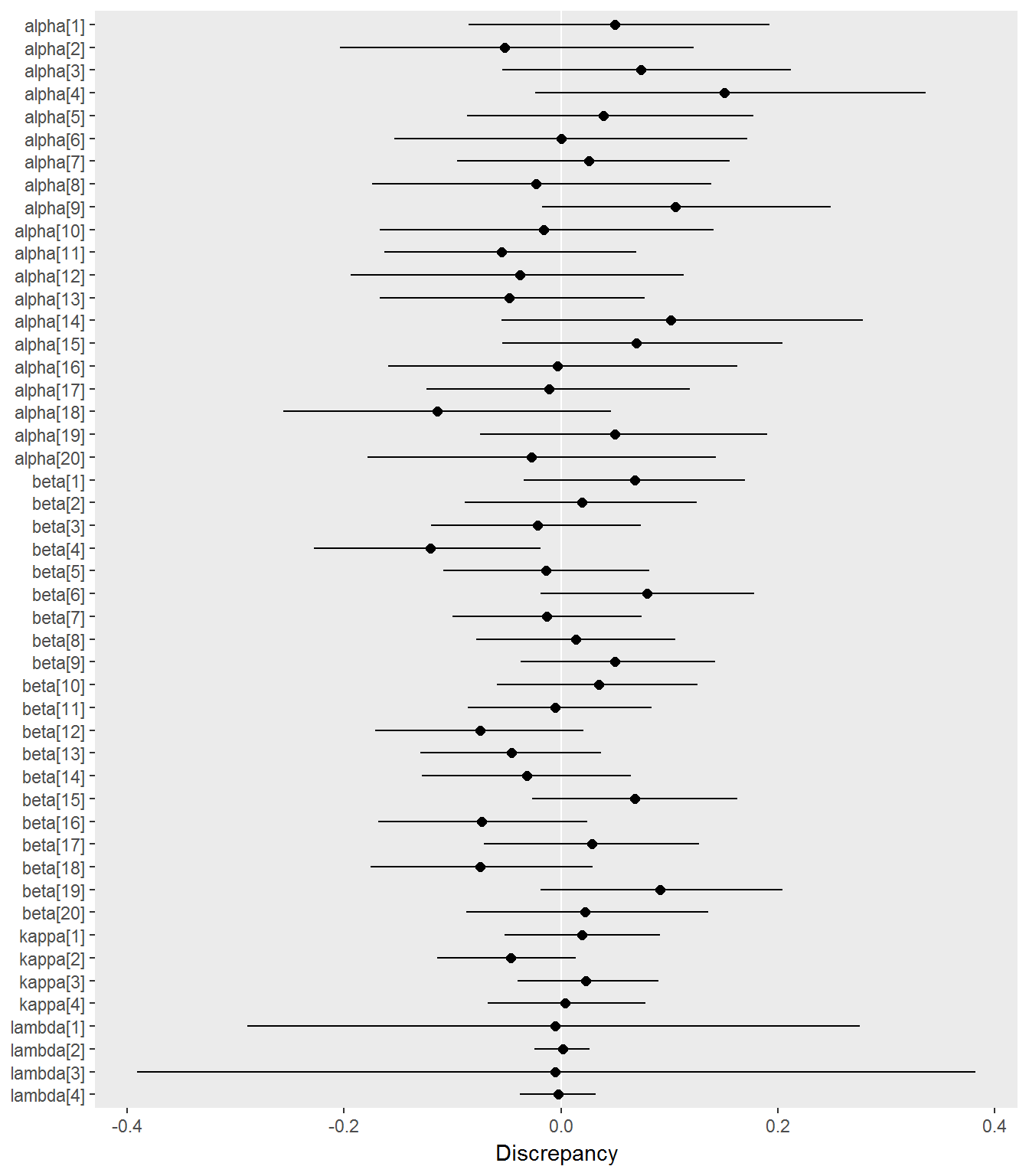Discrepancies between estimated and generating parameters. Points indicate the difference between the posterior means and generating values for a parameter, and horizontal lines indicate 95% posterior intervals for the difference. Most of the discrepancies are about zero, indicating that Stan successfully recovers the true parameters.

# 3 Example application

## 3.1 Data

The example data are from the Consumer Protection and Perceptions of Science and Technology section of the 1992 Euro-Barometer Survey (Karlheinzand and Melich 1992). Because these data do not include person covariates, the latent regression aspect of the model will include an intercept only.

# Convert the example dataset to an integer matrix with values 0 ... 3
M <- matrix(NA, ncol = ncol(Science), nrow = nrow(Science))
for(i in 1:ncol(M)) M[, i] <- as.integer(Science[, i]) - 1

The dataset contains 7 items and 392 persons with no missing responses. The items pertain to attitudes towards science and technology, and responses are scored on a 4-point Likert scale. For example, the text of the first item reads, “Science and technology are making our lives healthier, easier and more comfortable.” The response options are strongly disagree, disagree, agree, and strongly agree.

Before fitting the model, the response frequencies for each item are considered.

# Frequencies for each item
freqs <- t(apply(M, 2, table))
rownames(freqs) <- names(Science)
freqs
##              0   1   2   3
## Comfort      5  32 266  89
## Environment 29  90 145 128
## Work        33  98 206  55
## Future      14  72 210  96
## Technology  18  91 157 126
## Industry    10  47 173 162
## Benefit     21 100 193  78

The data are now formatted into a data list.

# Assemble data list for Stan
ex_list <- irt_data(M)

## 3.2 Rating scale model results

The data list is used to fit the rating scale model.

# Run Stan model
ex_rsm_fit <- irt_stan(ex_list, "rsm_latent_reg.stan", chains = 4, iter = 300)

As discussed above, convergence of the chains is assessed for every parameter, and also the log posterior density, using $$\hat{R}$$.

# Plot of convergence statistics
stan_columns_plot(ex_rsm_fit)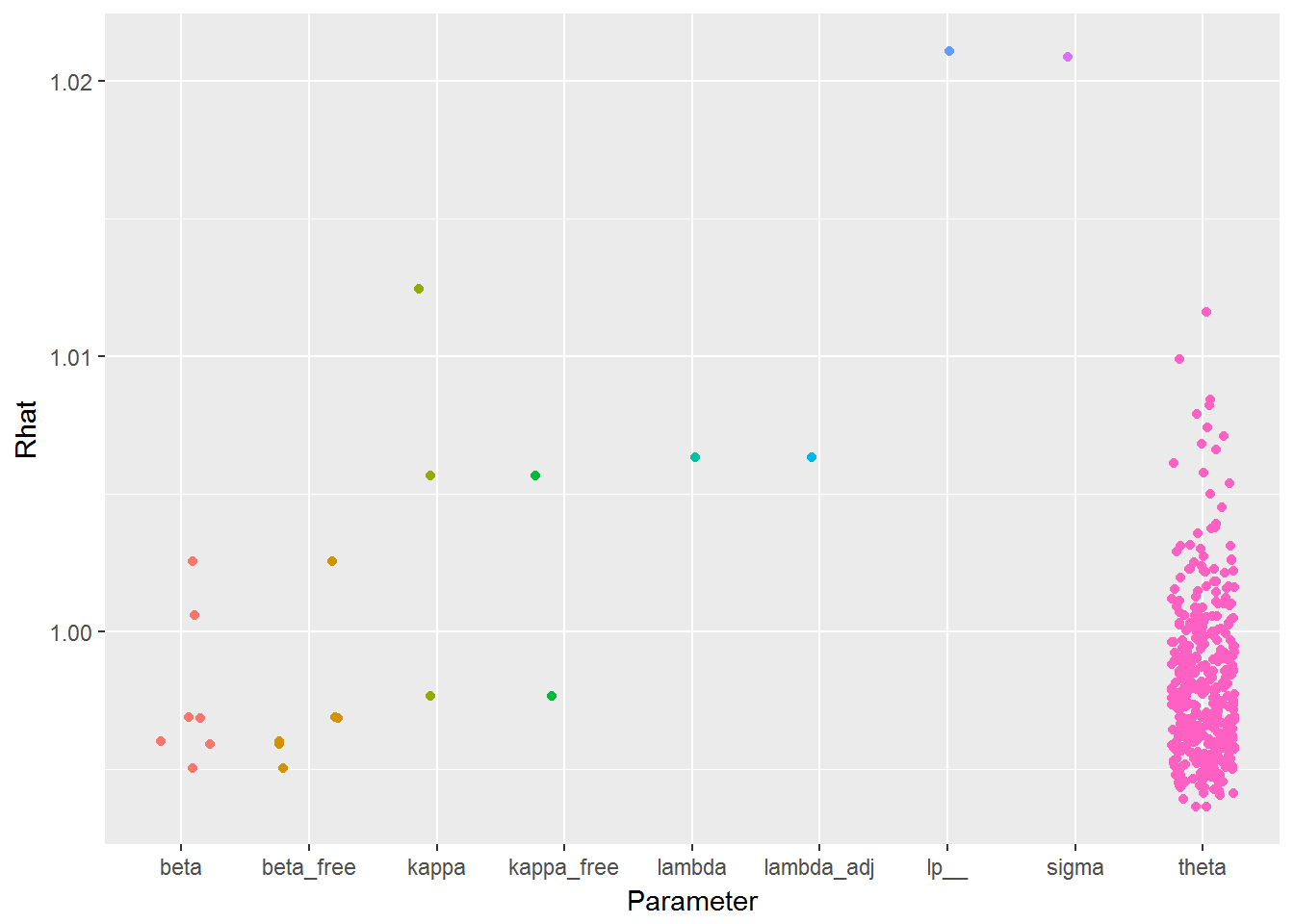Convergence statistics ($$\hat{R}$$) by parameter for the example. All values should be less than 1.1 to infer convergence.

Next we view a summary of the parameter posteriors.

# View table of parameter posteriors
print_irt_stan(ex_rsm_fit, ex_list)
## Inference for Stan model: rsm_latent_reg.
## 4 chains, each with iter=300; warmup=150; thin=1;
## post-warmup draws per chain=150, total post-warmup draws=600.
##
##              mean se_mean   sd  2.5%   25%   50%   75% 97.5% n_eff Rhat
## Item 1
##   beta   -0.26    0.00 0.07 -0.39 -0.30 -0.25 -0.21 -0.12   600 1.00
## Item 2
##   beta    0.07    0.00 0.07 -0.07  0.02  0.07  0.11  0.20   600 1.00
## Item 3
##   beta    0.46    0.00 0.06  0.34  0.42  0.46  0.50  0.58   600 1.00
## Item 4
##   beta    0.00    0.00 0.06 -0.12 -0.04  0.00  0.04  0.12   600 1.00
## Item 5
##   beta   -0.02    0.00 0.06 -0.14 -0.06 -0.02  0.02  0.10   600 1.00
## Item 6
##   beta   -0.51    0.00 0.07 -0.65 -0.56 -0.52 -0.46 -0.39   600 1.00
## Item 7
##   beta    0.27    0.00 0.06  0.15  0.22  0.27  0.31  0.38   462 1.00
## Rating scale step parameters
##   kappa  -1.13    0.01 0.08 -1.29 -1.19 -1.13 -1.08 -0.97   221 1.01
##   kappa  -0.37    0.00 0.06 -0.48 -0.40 -0.37 -0.32 -0.25   600 1.00
##   kappa   1.50    0.01 0.06  1.39  1.46  1.50  1.54  1.62   136 1.01
## Ability distribution
##   lambda  0.73    0.00 0.05  0.64  0.69  0.72  0.76  0.82   217 1.01
##   sigma      0.54    0.01 0.05  0.44  0.51  0.54  0.57  0.63    72 1.02
##
## Samples were drawn using NUTS(diag_e) at Mon Mar 06 14:14:54 2017.
## For each parameter, n_eff is a crude measure of effective sample size,
## and Rhat is the potential scale reduction factor on split chains (at
## convergence, Rhat=1).

## 3.3 Generalized rating scale model results

The data list is used to fit the generalized rating scale model.

# Run Stan model
ex_grsm_fit <- irt_stan(ex_list, "grsm_latent_reg.stan", chains = 4, iter = 300)

As discussed above, convergence of the chains is assessed for every parameter, and also the log posterior density, using $$\hat{R}$$.

# Plot of convergence statistics
stan_columns_plot(ex_grsm_fit)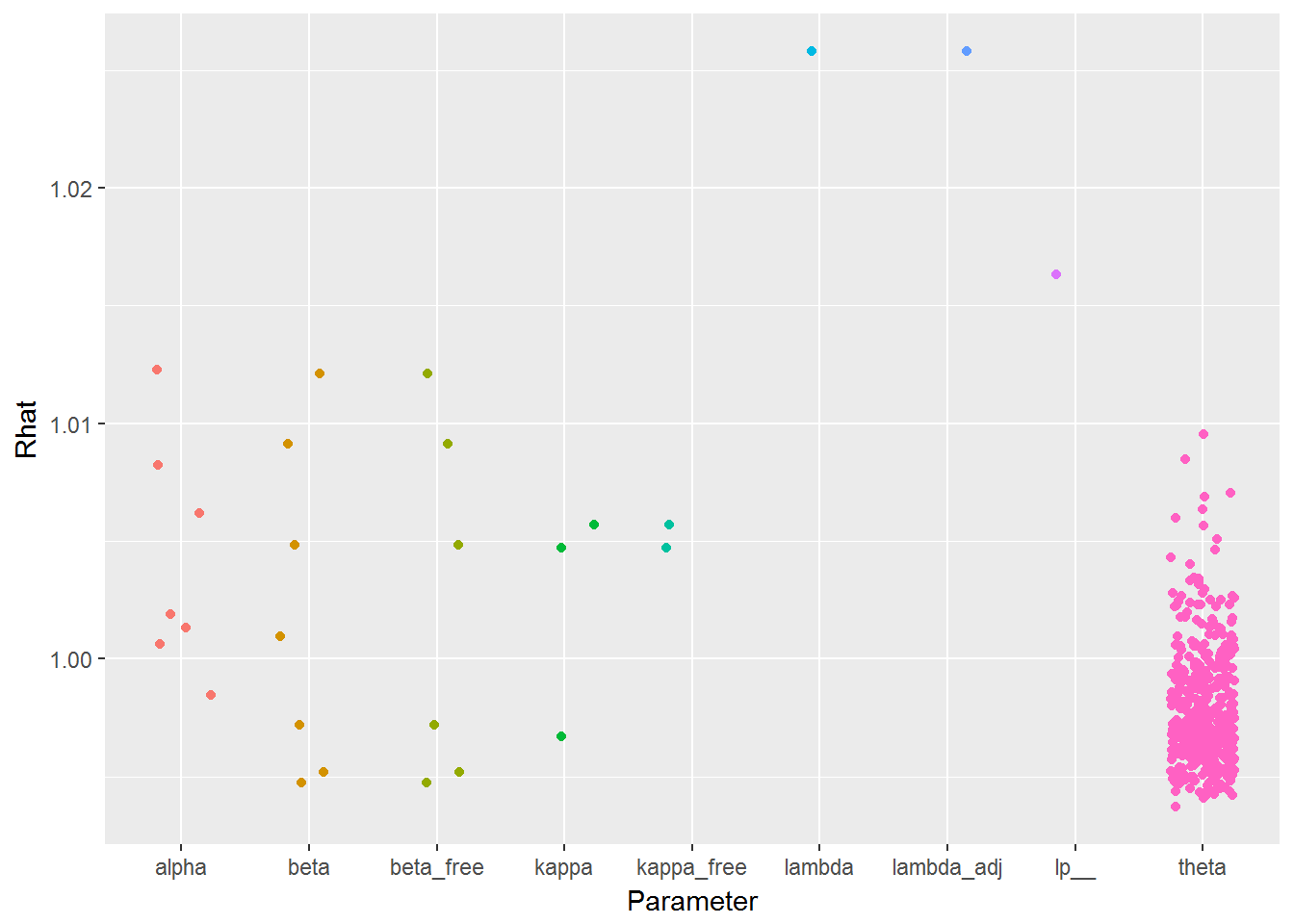Convergence statistics ($$\hat{R}$$) by parameter for the example. All values should be less than 1.1 to infer convergence.

Next we view a summary of the parameter posteriors.

# View table of parameter posteriors
print_irt_stan(ex_grsm_fit, ex_list)
## Inference for Stan model: grsm_latent_reg.
## 4 chains, each with iter=300; warmup=150; thin=1;
## post-warmup draws per chain=150, total post-warmup draws=600.
##
##              mean se_mean   sd  2.5%   25%   50%   75% 97.5% n_eff Rhat
## Item 1
##   alpha   0.29    0.00 0.08  0.14  0.23  0.28  0.34  0.45   481 1.00
##   beta   -0.63    0.00 0.11 -0.84 -0.71 -0.63 -0.54 -0.41   600 1.00
## Item 2
##   alpha   1.32    0.01 0.14  1.06  1.23  1.32  1.41  1.61   227 1.00
##   beta    0.81    0.01 0.16  0.51  0.70  0.80  0.91  1.11   370 1.01
## Item 3
##   alpha   0.14    0.00 0.06  0.04  0.09  0.13  0.17  0.27   600 1.00
##   beta   -0.07    0.00 0.09 -0.23 -0.13 -0.07  0.00  0.12   600 1.00
## Item 4
##   alpha   0.23    0.00 0.08  0.09  0.18  0.23  0.29  0.40   373 1.01
##   beta   -0.43    0.01 0.12 -0.67 -0.52 -0.43 -0.35 -0.20   466 1.01
## Item 5
##   alpha   1.13    0.01 0.13  0.89  1.04  1.13  1.21  1.37   394 1.01
##   beta    0.52    0.01 0.14  0.26  0.43  0.52  0.61  0.79   465 1.00
## Item 6
##   alpha   1.10    0.01 0.13  0.84  1.01  1.10  1.20  1.37   292 1.01
##   beta   -0.05    0.01 0.14 -0.32 -0.13 -0.05  0.04  0.22   600 0.99
## Item 7
##   alpha   0.22    0.00 0.08  0.09  0.17  0.21  0.27  0.39   600 1.00
##   beta   -0.16    0.00 0.11 -0.36 -0.23 -0.17 -0.10  0.07   600 1.00
## Rating scale step parameters
##   kappa  -1.23    0.00 0.08 -1.41 -1.28 -1.23 -1.17 -1.08   600 1.01
##   kappa  -0.39    0.00 0.06 -0.50 -0.43 -0.39 -0.35 -0.27   600 1.00
##   kappa   1.62    0.00 0.06  1.50  1.58  1.62  1.66  1.75   600 1.00
## Ability distribution
##   lambda  1.26    0.01 0.10  1.05  1.19  1.26  1.33  1.45   127 1.03
##
## Samples were drawn using NUTS(diag_e) at Mon Mar 06 14:15:49 2017.
## For each parameter, n_eff is a crude measure of effective sample size,
## and Rhat is the potential scale reduction factor on split chains (at
## convergence, Rhat=1).